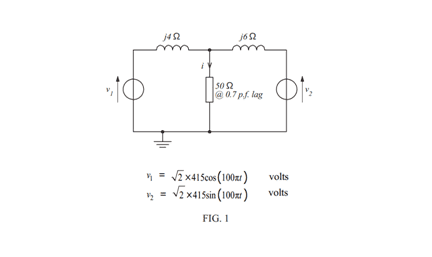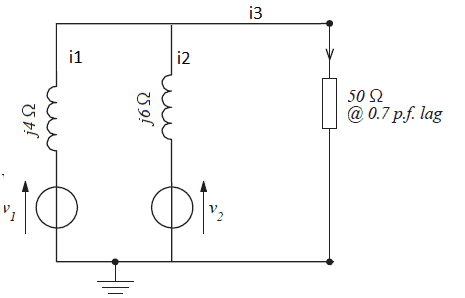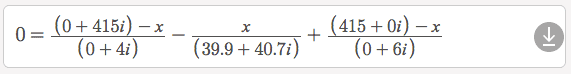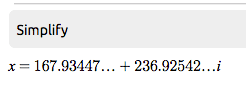# Thevenin's Theorem, Superposition & Norton's Theorem

## Homework Statement

FIGURE 1 shows a 50 Ω load being fed from two voltage sources via
their associated reactances. Determine the current i flowing in the load by:

(a) applying Thévenin’s theorem
(b) applying the superposition theorem
(c) by transforming the two voltage sources and their associated
reactances into current sources (and thus form a pair of Norton
generators)## The Attempt at a Solution

(a)
Converting V1 & V2 to RMS values and V1 to a sin value:
V1 = 415sin(100πt+90)
V2 = 415sin(100πt)
and from Asin(ωt+Φ)
V1 = 415∠90V or 0+j415 V
V2 = 415∠0V or 415+j0 V

Then current around the circuit:
I =V/Z = (V2-V1) / (Z2+Z1) = (415-j415) / (j10)
This as a polar form division:
586.8986∠-45 / 10∠90 = 58.68986∠-135 A or -41.5-j41.5

Vt = V2 - Z2*I = 415+j0 - (0+j6)(-41.5-j41.5) = 415+j0-(-j249-249j^2) as j^2 = -1 then:
Vt = 415-(249-j249) = 166+j249V

ZL = 50@0.7lag = 50∠-45.572998 = 35+j35.70714Ω
Zt = j4in parallel with j6= j2.4
I = V / ZL + Zt = 166+j249 / ( 0+j2.4 + 35+j35.70714 ) = 166+j249 / 35+j38.10714
this as a polar division I = 299.26∠56.310 / 51.741∠47.434 = 5.784∠8.876A

I have only completed part (a), once I know I'm on the right track I'll complete b & c. All help is greatly appreciated as always.

#### Attachments

Jason-Li, Just wondering here...for your calculation for figuring out the current around the circuit, does that still hold up for the load being a short? Open? Dan

Jason-Li, Just wondering here...for your calculation for figuring out the current around the circuit, does that still hold up for the load being a short? Open? Dan

Hi dvscrobe, I am not going to lie to you this circuit is confusing me, I'll give it a bash though. I have redrawn (on paint) the circuit like below. So if I were to say that the load is shorted then could I use KCL so that:
I3 = I1+I2
= 415∠90/ / 4∠90 + 415∠0 / 6∠90
= 103.75∠0 + 69.167∠-90
= 103.75-j69.167A

Which is different to my calculated -41.5-j41.5 A but I could then do
Vab = I3 * Zt
=(103.75-j67.167) * j2.4
= 166+j249
Which is the same as my earlier Vab figure?
All help is much appreciated.#### Attachments

Jason-Li, I liked how you simplified things up a little to start the problem. Finding the RMS value, phase shift, and frequency was pretty easy. When you were trying to calculate the current in the outer loop, that won't work. When going around the outer loop, first loop, and second loop, you can use Kirchoff's Voltage Law by summing the voltage sources and voltage drops. For currents, consider the nodes. You have one on the top part of the load and one on the bottom part of the load. Current in must equal current out. Kind of like a current differential relay in a substation. You essentially have 3 current variables. I1 though the first voltage source, I2 through the second voltage source, and I3 through the load. I1 & I2 basically sums up to go into the load and becomes I3. It takes a little algebra to get it. Dan

Hi Dan,

I'm not sure what loops you are meaning when you say I can't calculate that way? If I1 & I2 are from V1 & V2 respectively does that not mean that I3 is the current in the load as I3 goes into the node prior to the load hence must leave it?

Also if it isn't possible to do this unsure of why The value of Vab worked out the same on both methods?

Thanks for the help.

Dan, I see what you mean so I could treat it sort of like mesh analysis, so if I redo it as follows:
Assuming all currents are clockwise (for ease)
Loop 1:
V1-Z1*I1-ZL(I1-I2)=0
V1-Z1*I1-ZL*I1+Zl*I2
V1=Z1*I1+ZL*I1-ZL*I2
V1=I1(Z1+ZL)-ZL*I2
V1=I1(j4+35+j35.707)-I2(35+j35.707)
V1=I1(35+j39.707)-I2(35+j35.707)

Loop 2:
-I2*Z2-V2-ZL(I2-I1)=0
-I2*Z2-V2-I2*ZL-I1*ZL=0
-I2(Z2+ZL)-V2-I1*ZL=0
V2=I2(Z2+ZL)+I1*ZL
V2=I2(35+j41.707)+I1(35+j35.707)

So:
V1=I1*Z1+I1*ZL-I2*Z2

V2=I2*Z2+I2*ZL+I1*ZL

V1-V2=I1*Z1+I1*ZL-I2*Z2 - (I2*Z2+I2*ZL+I1*ZL)
=I1*Z1-2*I2*Z2-I2*Z2

Not going to lie I am having a hard time doing the simultaneous equation? I would do another loop for the outside but I thought meshes shouldn't have other loops in them?

If I were to treat the load like a short:

V1-Z1*I1-I1+I2)=0
-I2*Z2-V2-I2+I1)=0

V1=I1*Z1+I1-I2
V2=-I2*Z2-I2+I1

V2=-I2*Z2-I2+I1
V2=I1+I2(-Z2-1)
V2-I1=I2(-Z2-1)
I2=(V2-I1) / (-Z2+1)

V1=I1*Z1+I1-[(V2-I1) / (-Z2+1)]
I1= (V1+V2-Z2*V1) / (-Z2(Z1+1)+Z1+2)
I1=(j415+415-j6*j415) / (-j6(j4+1)+j4+2)
=109.706+j24.412 A

I2=(V2-I1) / (-Z2+1)
I2=(415-109.706-j24.412) / (-j6+1)
I2=12.2099+j48.847 A

109.706+j24.412 - (12.2099+j48.847) = 97.4961-j24.435A

This is completely different and I have somewhat confused myself haha.

Hi Dan, I know its a lot of reading, but is the following method okay? (if so ignore the previous 2 posts)

Thevenin equivalent reactance: j2.4

With V30 being the centre node:
(v1-V30)/Z1+(V2-V30)/Z2=0
V30=(V1*z2+v20*Z1)/Z1+z2)
= (j415*j6+415*j4)/(j4+j6)
=166+j249
I=V/(Zt+ZL)
=(166+j249) / (j2.4+35+j35.10714)
= 5.784∠8.876A

This is using nodal analysis and I still get the same answer? Does this mean that the answer is proved or am I so far off the mark its ridiculous?

Jason-Li, I took at a stab at this and it may be time to call in the experts. I don't think I got the correct answer. Sorry. Works much better with DC circuits. I use a TI-86 that lets me plug values into a variable. So a letter with a box around it is a variable that I programmed. Unfortunately, my current from the 2nd voltage source on the right is higher in magnitude than the current from the 1st voltage source on the left. Your problem has a higher inductive reactance on the right side (j6) than that of left side (j4). Hopefully, someone else can chime in, cause now I got myself wondering. Dan

#### Attachments

• 2019-01-20 15-49.pdf
841.1 KB · Views: 166
• 2019-01-20 15-50.pdf
328.3 KB · Views: 146
scottdave
Homework Helper
I've looked through it a couple of times and I don't see where you calxulate the Thevenin impedance = (open ckt voltage) ÷ (short ckt current).

I'm on my phone, so maybe I missed it. It looks like the purpose of this exercise is for you to calculate it 3 different ways, and if you did it correctly, then you'll arrive at the same answer.
It also exposes to different methods, because some methods are better suited for some circuits than others.

Hi scottdave,

I calculated the Zt by calculating Z1 & Z2 as in parallel as the voltage sources won't have impedences. Hence Zt = J2.4.

Pretty certain thats correct?

I'm with you there. You just calculated the Thevenin resistance. I agree with (0+j2.4). Dan

scottdave
Homework Helper
Ok I did find where you showed Zt as j6 in parallel with j4Ω is j2.4Ω. I confirm the same value doing it the way I learned (open ckt voltage) ÷ (short ckt current).

Ok thanks.

Does the rest of it look like correct methodology & answer to you?

Put me and Dan out of our misery haha.

I'll start working on the superposition theorem anyways.

scottdave
Homework Helper
I am still on my phone. I haven't taken a look at those PDF documents yet. But I confirm the calculations to get 5.784 A ∠8.876° in reference to source V2. This is important to specify. Somebody else might do all of the calculations using V1 as the reference.

Scottdave,

So would I reform it as follows?

I =√2*5.784sin(100πt+8.876)

Hence showing the reference point? or should I lose the root 2?

scottdave
Homework Helper
If I'm using the cosine or sine notation, then I would show the peak amperes multiplied by sine. So I would multiply by the root 2 in that case.

So I could write either

I =√2*5.784sin(100πt+8.876) A
or
I =8.180sin(100πt+8.876) A

•scottdave
Okay I have done the following for superposition:
For V1 acting alone:
Current from V1: V1/(z1+Z2) = j415/j10 = 41.5A
Vab(V1)=41.5*Z2 = 41.5*j6 = j249V
For V2 acting alone:
Current from V2 = 415 / j10 = -41.5A
Vab(V2) = V2 - (-j41.5)*Z2 = 415 + j41.5*j6 = 166V

Superposition of Vab(V1) & Vab(V2) = 166 + j249
current is calculated the same was as previous.

•scottdave
Jason-Li, Are you given the answer for this problem? I am interested in knowing the confirmed answer when you get it. Thanks. Dan.

Dan, sadly not, I am relatively confident however of that answer as I got it for all 3 methods. Cheers.

•scottdave
scottdave
Homework Helper
Jason-Li, Are you given the answer for this problem? I am interested in knowing the confirmed answer when you get it. Thanks. Dan.
Yes, me too. I have a high confidence level, but would like the confirmation, since it's been a little while

All, here is another way of going about this. The Nodal method basically says to model the currents leaving or entering a particular node, in this case the node on top of the load, which I call here as V3. If a current is entering a node, it is positive. If leaving, it is negative. Summing all the currents should equal zero. It is fair to assume for the moment that V1 provides a current into the node, V2 provides a current into the node, and then both of these current sum and leave the node, going to the load. If you convert the currents in its equivalent Ohm's Law of voltage over impedance, you will be left with an equation that has only one variable to solve. It's a lot of algebra to isolate it, but you could use Symbolab.com to crunch complex numbers and just let Symbolab solve for V3 or x in this equation solver. Of course, you won't likely be able to use Symbolab during a test.x is found as 167.9+236.9i.If you take this voltage and divide it by the impedance of the load, you get a load current of 5.0 + .8i

#### Attachments

•scottdave